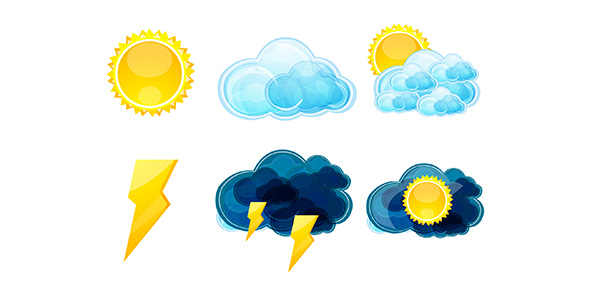# Science Simester Exam

28 QuestionsSettingsThis test is on all the stuff in the planetary forcaster book for the simester exam

Related Topics
• 1.
Explain the difference between direct sunlight and indirect sunlight.  When writing the answer address these questions:~Which type of sunlight absorbs more heat energy and which one reflects more heat energy?~Which type of sunlight is at a 90 degree angle and which is at any other angle except 90 degrees?
• 2.
• 3.
• 4.
Draw a picture of the water cycle on a seperate sheet of paper.
• 5.
A measur of the average amount of kinetic energy found in the molecules of a substance
• A.

Temperauture

• B.

Visualization

• C.

Increased elevation

• 6.
Air temperatures measured around 4ft 1in - 6ft 7in above the ground
• A.

Kinetic energy

• B.

Surface temperature

• C.

Potential evergy

• 7.
Shows a picture of the information
• A.

Temperature

• B.

Air

• C.

Visualization

• 8.
______________is the energy of moving objects.
• 9.
_________________is the potential energy of an object.
• 10.
I will go to the mall and eat lots of candy during winter break.  This is called a________.
• 11.
• 12.
Does air have mass?
• A.

Yes, because without mass there would be no air and we would not exist

• B.

Yes, because without mass there would be air and we would exist

• C.

Yes, because without mass there would be dogs and we would not exist

• 13.
• A.

Temperature

• B.

Biome

• C.

Relative humidity

• 14.
Measures wind speed
• A.

Thermometer

• B.

Biome

• C.

Anemometer

• 15.
Gives meteorologists info about the temperature of the air
• A.

Thermometer

• B.

Biome

• C.

Revolution

• 16.
A__________ is a planets journey around the sun.A__________is the time it takes for a planet to make one full turn around its axis.
• 17.
The________ ___________ is the day with the least number of daylight hours.The_________ __________is the day with the greatest number of daylight hours.
• 18.
The amount of evergy required to raise the temperature of 1 gram of a substance by 1 degree celsius
• A.

Convection

• B.

Specific heat capacity

• C.

Increased elevation

• 19.
Movement of heat energy from one location to another as a result of the movement of particles that are storing that energy
• A.

Decreased elevation

• B.

Pressure

• C.

Convection

• 20.
Increased elevation = ____________pressure and _________temperature.Decreased elevation = ___________pressure and  _________temperature.
• 21.
There is _________pressure at the summit then at the bottom of a mountain.There is _________pressure at the bottom that at the summit of a mountain.
• 22.
• A.

Less dense than the air around it

• B.

Less molecules that have hats than the air around it

• C.

Has more petunias

• 23.
• 24.
Inch is to length as __________________ is to temperature.Pound is to weight as _________________is to heat energy.
• 25.
The area where two air masses collide
• A.

Front

• B.

Occluded front

• C.

Stationary front

• 26.
• A.

Stationary front

• B.

Front

• C.

Occluded front

• 27.
• A.

Occluded front

• B.

Stationary front

• C.

Front

• 28.
________ _________ are winds that blow near earth's surface and over short distances._________ ________ is the movement of air between the north and south poles and equater and spans over the whole earth and @ higher altitudes.